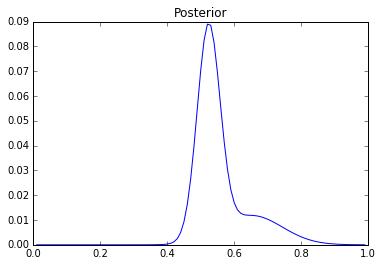### Bayesian Inference Intro¶

Let's proceed with the coin tossing example. We have to formalize our prior. Let to it like this:

In :
%matplotlib inline
from numpy import *
from scipy import stats
from matplotlib.pyplot import *
from numpy.linalg import norm

t = arange(.01, 1, .01)

prior = .1*stats.norm.pdf(t-.5, loc=0, scale=1)+.9*stats.norm.pdf( (t-.5), loc=0, scale=0.03500)
prior /= sum(prior) #Make everything sum up to 1
figure(1)
plot(t,prior)
title("Prior")

/usr/lib/python2.7/dist-packages/matplotlib/font_manager.py:273: UserWarning: Matplotlib is building the font cache using fc-list. This may take a moment.
warnings.warn('Matplotlib is building the font cache using fc-list. This may take a moment.')

Out:
<matplotlib.text.Text at 0x7f9ccd980ad0>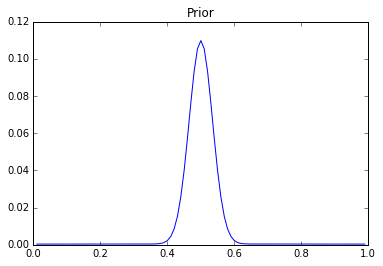This prior indicates that we think that the coin is basically fair, with maybe a slight deviation from 50%. Let's see what happens if we toss the coin 100 times, and get 60 heads.

In :
figure(2)

N = 60
likelihood = (t**N)*((1-t)**(100-N))

plot(t, likelihood)
title("Likelihood")

Out:
<matplotlib.text.Text at 0x7f9ccb8799d0>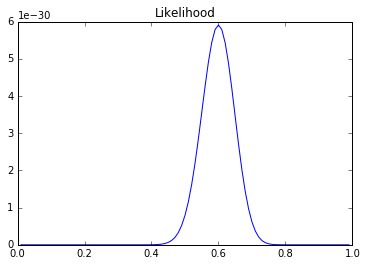Now, we can look at the posterior:

In :
posterior = likelihood*prior
figure(3)
plot(t, posterior)
title("Posterior")

Out:
<matplotlib.text.Text at 0x7f9ccb812750>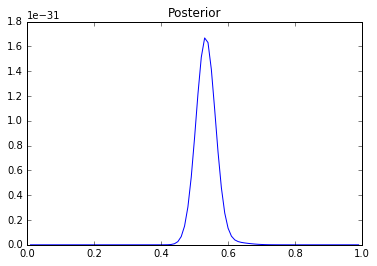What if we got 99 heads out of 100 tries?

In :
N = 100
likelihood = (t**N)*((1-t)**(100-N))
figure(2)
plot(t, likelihood)
title("Likelihood")

posterior = likelihood*prior
posterior /= sum(posterior)
figure(3)
plot(t, posterior)
title("Posterior")

Out:
<matplotlib.text.Text at 0x7f9ccb6ba6d0>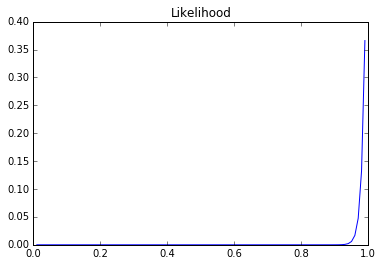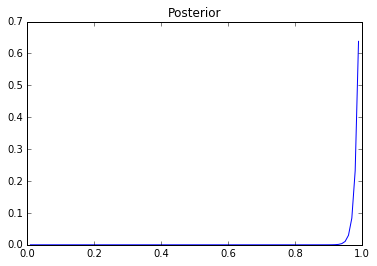What about 10 out of 10?

In :
N = 10
likelihood = (t**N)*((1-t)**(10-N))
figure(2)
plot(t, likelihood)
title("Likelihood")

posterior = likelihood*prior
posterior /= sum(posterior)
figure(3)
plot(t, posterior)
title("Posterior")

Out:
<matplotlib.text.Text at 0x7f9ccb522e90>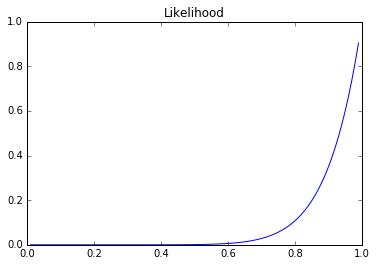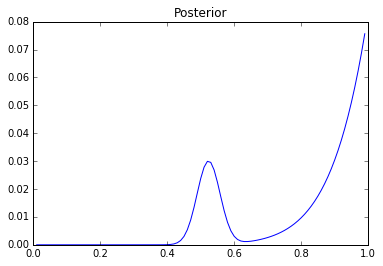Do we believe that the coin could be so biased? Maybe not. In that case, our prior probability was wrong. Let's make the "wide" Gaussian narrower.

In :
prior = .1*stats.norm.pdf(t-.5, loc=0, scale=.1)+.9*stats.norm.pdf( (t-.5), loc=0, scale=0.03500)
prior /= sum(prior) #Make everything sum up to 1
figure(1)
plot(t,prior)
title("Prior")

Out:
<matplotlib.text.Text at 0x7f9ccb5a93d0>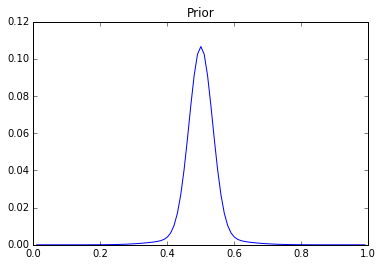In :
N = 10
likelihood = (t**N)*((1-t)**(10-N))
figure(2)
plot(t, likelihood)
title("Likelihood")

posterior = likelihood*prior
posterior /= sum(posterior)
figure(3)
plot(t, posterior)
title("Posterior")

Out:
<matplotlib.text.Text at 0x7f9ccb5be8d0>